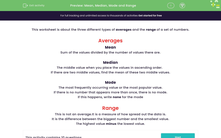# Mean, Median, Mode and Range

In this worksheet, students find the mean, median, mode and range of the given sets of numbers.Key stage:  KS 3

Curriculum topic:   Statistics

Curriculum subtopic:   Understand Variables, Representation, Measures and Spread

Difficulty level:#### Worksheet Overview

This worksheet is about the three different types of averages and the range of a set of numbers.

Averages

Mean

Sum of the values divided by the number of values there are.

Median

The middle value when you place the values in ascending order.

If there are two middle values, find the mean of these two middle values.

Mode

The most frequently occurring value or the most popular value.

If there is no number that appears more than once, there is no mode.

If this happens, write none for the mode

Range

This is not an average.It is a measure of how spread out the data is.

It is the difference between the biggest number and the smallest value.

The highest value minus the lowest value.

### What is EdPlace?

We're your National Curriculum aligned online education content provider helping each child succeed in English, maths and science from year 1 to GCSE. With an EdPlace account you’ll be able to track and measure progress, helping each child achieve their best. We build confidence and attainment by personalising each child’s learning at a level that suits them.

Get started